# Key Signatures Quiz

To give you some practice with key signatures, here are some questions. Click the "check answer" button to see if you answered correctly.

1. What key signature is this?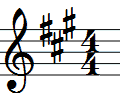check answer

Correct!

Incorrect.

2. What key signature is this?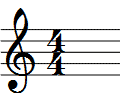check answer

Correct!

Incorrect.

3. What key signature is this?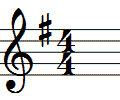check answer

Correct!

Incorrect.

4. What key signature is this?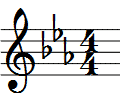check answer

Correct!

Incorrect.

5. What key signature is this?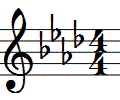check answer

Correct!

Incorrect.

6. What key signature is this?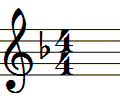check answer

Correct!

Incorrect.

7. What key signature is this?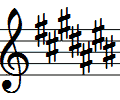check answer

Correct!

Incorrect.

8. What key signature has 3 flats?

check answer

Correct!

Incorrect.

9. What key signature is this?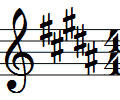check answer

Correct!

Incorrect.

10. What key signature is this?check answer

Correct!

Incorrect.

11. What key signature is this?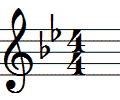check answer

Correct!

Incorrect.

12. What key signature is this?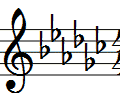check answer

Correct!

Incorrect.

13. How many sharps are in the key of F sharp?

check answer

Correct!

Incorrect.

14. What key signature is this?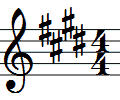check answer

Correct!

Incorrect.

15. What key signature is this?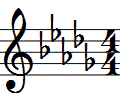check answer

Correct!

Incorrect.

16. What key signature is this?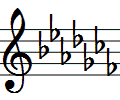check answer

Correct!

Incorrect.

17. How many sharps are in the key of F?

check answer

Correct!

Incorrect.

18. What key signature is this?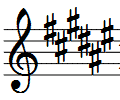check answer

Correct!

Incorrect.

19. What key signature has 4 flats?

check answer

Correct!

Incorrect.

20. How many sharps does the key of G have?

check answer

Correct!

Incorrect.